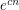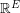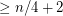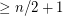# Exponentially many perfect matchings in cubic graphs (Solved)

 Importance: High ✭✭✭
 Author(s): Lovasz, Laszlo Plummer, Michael D.
 Subject: Graph Theory » Basic Graph Theory » » Matchings
 Keywords: cubic perfect matching
 Posted by: mdevos on: June 30th, 2008
 Solved by: L. Esperet, F. Kardoš, A. D. King, D. Král', S. Norine. Exponentially many perfect matchings in cubic graphs. Advances in Mathematics, to appear, 2011. DOI: 10.1016/j.aim.2011.03.015
Conjecture   There exists a fixed constantso that every-vertex cubic graph without a cut-edge has at leastperfect matchings.

A classical theorem of Petersen [P] asserts that every cubic graph without a cut-edge has a perfect matching (nowadays this is usually derived as a corollary of Tutte's 1-factor theorem). In the 70's, Lovasz and Plummer made the above conjecture, which asserts that every such graph has exponentially many perfect matchings. Despite considerable work on the topic of perfect matchings, this conjecture remains open.

Letbe anvertex cubic graph. Edmond's matching polytope theorem gives a precise description of what vectors incan be written as a convex combination of characteristic vectors of perfect matchings. Assuming this polytope is nonempty, the number of perfect matchings inis at least its dimension plus one. Using this technique, it can be shown (see [ELP] and [N]) thathasmatchings if it has no cut-edge, andperfect matchings if it is cyclically 4-edge-connected. Similar bounds can be achieved using Lovasz' matching lattice theorem [L]. Kral, Sereni, and Stiebitz [KSS] improved the lower bound for graphs without a cut-edge to. In fact, these authors do much more: they list all 17 of the-vertex cubic graphs which have fewer thanperfect matchings. More recently, Esperet, Kardos, and Kral [EKK] proved that bridgeless cubic graphs have a superlinear number of perfect matchings.

There are two significant classes of cubic graphs for which the conjecture is known: bipartite and planar. It was proved for bipartite graphs by Voorhoeve [V], and this was later extended to general regular bipartite graphs by Schrijver [S] (see also Gurvits [G] for an interesting alternate proof). Very recently, Chudnovsky and Seymour [CS] have proved the conjecture for planar graphs.

## Bibliography

[CS] M. Chudnovsky and P. Seymour. Perfect matchings in planar cubic graphs.

[EKK] L. Esperet, F. Kardos, D. Kral'. A superlinear bound on the number of perfect matchings in cubic bridgeless graphs.

[KSS] D. Kral, J.-S. Sereni, and M. Stiebitz, A new lower bound on the number of perfect matchings in cubic graphs.

[P] J. Petersen, Die Theorie der regularen graphs. Acta Math., 15(1):193–220, 1891.

[ELP] J. Edmonds, L. Lovasz, and W. R. Pulleyblank. Brick decompositions and the matching rank of graphs. Combinatorica, 2(3):247–274, 1982.

[G] L. Gurvits. Hyperbolic polynomials approach to Van der Waerden/Schrijver-Valiant like conjectures: sharper bounds, simpler proofs and algorithmic applications. In STOC’06: Proceedings of the 38th Annual ACM Symposium on Theory of Computing, pages 417–426, New York, 2006. ACM.

[L] L. Lovasz. Matching structure and the matching lattice. J. Combin. Theory Ser. B, 43(2):187–222, 1987.

[N] D. Naddef. Rank of maximum matchings in a graph. Math. Programming, 22(1):52–70, 1982.

[S] A. Schrijver. Counting 1-factors in regular bipartite graphs. J. Combin. Theory Ser. B, 72(1):122–135, 1998.

[V] M. Voorhoeve. A lower bound for the permanents of certain (0, 1)-matrices. Nederl. Akad. Wetensch. Indag. Math., 41(1):83–86, 1979.

* indicates original appearance(s) of problem.

### Conjecture proven

The conjecture has recently been proven by Esperet, Kardos, King, Kral and Norine. The paper is now being prepared for publication.

### Preprint up on arXiv

The paper is now available in preprint form on arXiv. http://arxiv.org/abs/1012.2878